# Презентация, доклад Molecular-kinetic theory of ideal gases

Вы можете изучить и скачать доклад-презентацию на тему Molecular-kinetic theory of ideal gases. Презентация на заданную тему содержит 33 слайдов. Для просмотра воспользуйтесь проигрывателем, если материал оказался полезным для Вас - поделитесь им с друзьями с помощью социальных кнопок и добавьте наш сайт презентаций в закладки!
Презентации» Физика» Molecular-kinetic theory of ideal gases

Слайды и текст этой презентации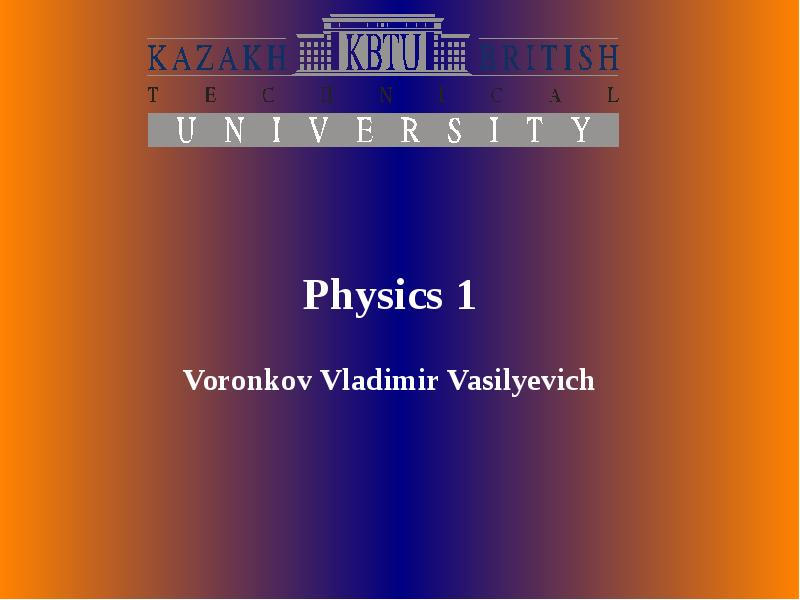Описание слайда: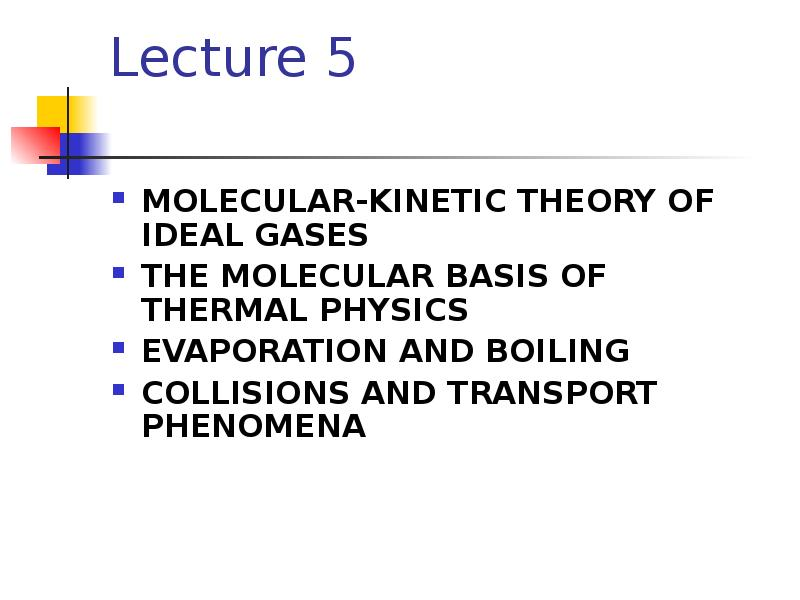Описание слайда:
Lecture 5 MOLECULAR-KINETIC THEORY OF IDEAL GASES THE MOLECULAR BASIS OF THERMAL PHYSICS EVAPORATION AND BOILING COLLISIONS AND TRANSPORT PHENOMENA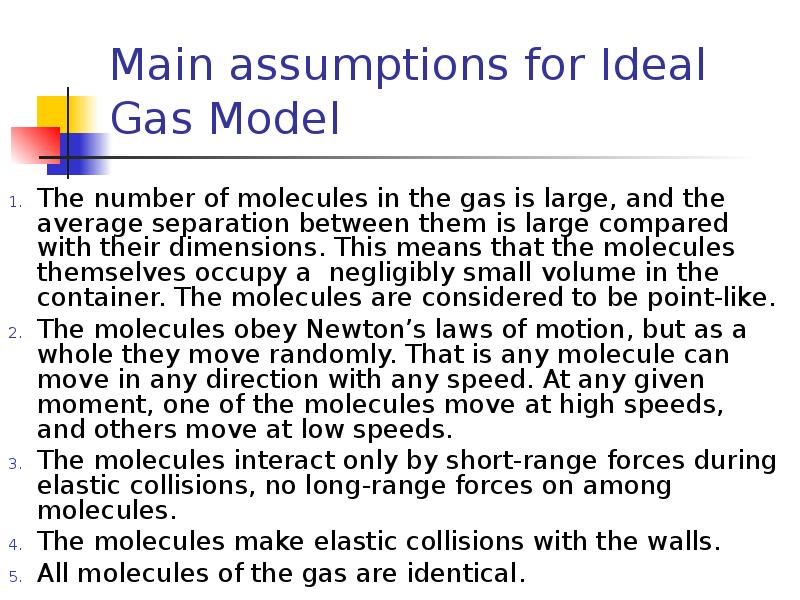Описание слайда:
Main assumptions for Ideal Gas Model The number of molecules in the gas is large, and the average separation between them is large compared with their dimensions. This means that the molecules themselves occupy a negligibly small volume in the container. The molecules are considered to be point-like. The molecules obey Newton’s laws of motion, but as a whole they move randomly. That is any molecule can move in any direction with any speed. At any given moment, one of the molecules move at high speeds, and others move at low speeds. The molecules interact only by short-range forces during elastic collisions, no long-range forces on among molecules. The molecules make elastic collisions with the walls. All molecules of the gas are identical.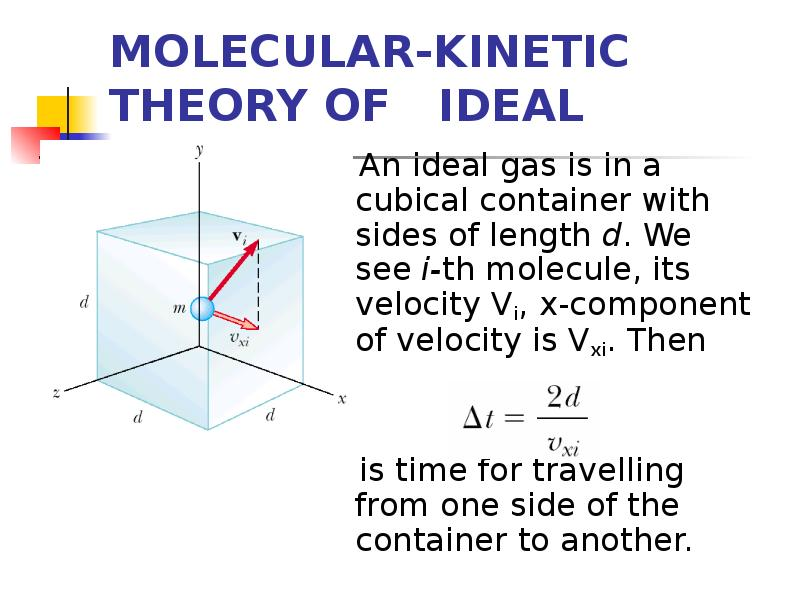Описание слайда:
MOLECULAR-KINETIC THEORY OF IDEAL GASES An ideal gas is in a cubical container with sides of length d. We see i-th molecule, its velocity Vi, x-component of velocity is Vxi. Then is time for travelling from one side of the container to another.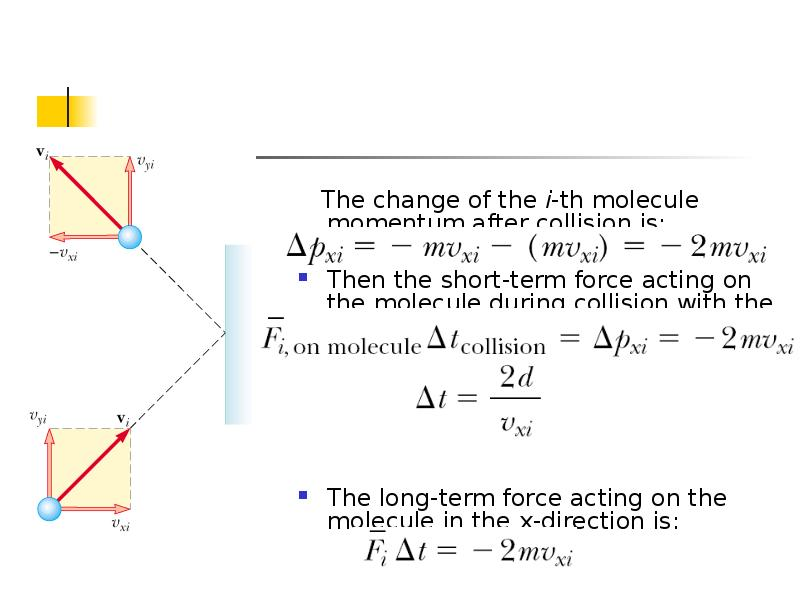Описание слайда:
The change of the i-th molecule momentum after collision is: Then the short-term force acting on the molecule during collision with the wall is: The long-term force acting on the molecule in the x-direction is: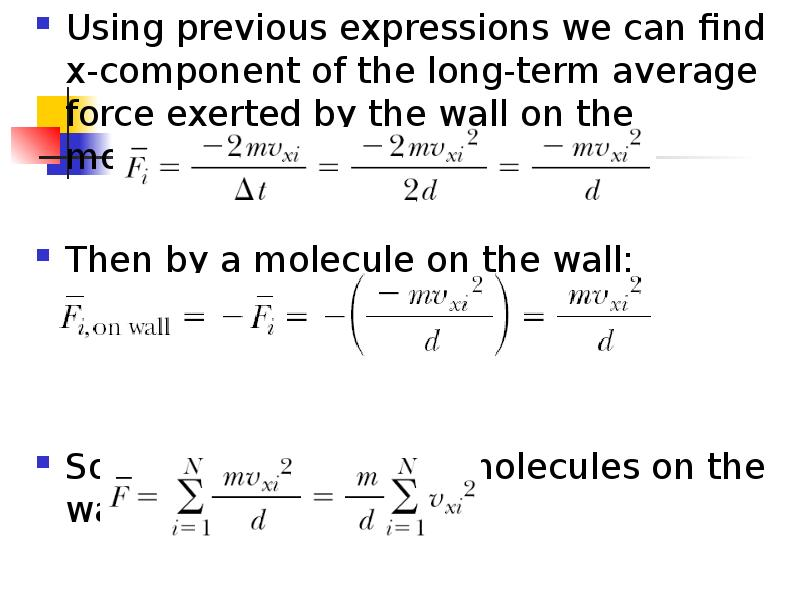Описание слайда:
Using previous expressions we can find x-component of the long-term average force exerted by the wall on the molecule as: Using previous expressions we can find x-component of the long-term average force exerted by the wall on the molecule as: Then by a molecule on the wall: So the net force of all molecules on the wall: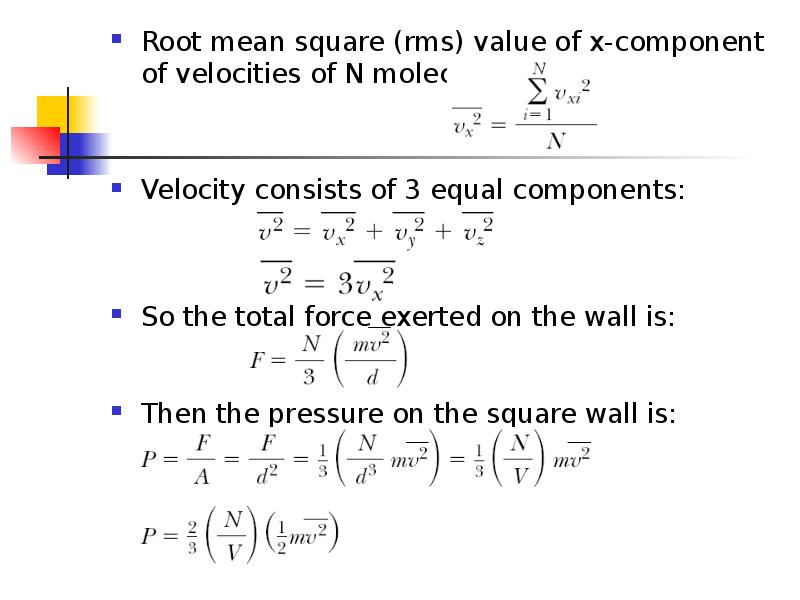Описание слайда:
Root mean square (rms) value of x-component of velocities of N molecules: Root mean square (rms) value of x-component of velocities of N molecules: Velocity consists of 3 equal components: So the total force exerted on the wall is: Then the pressure on the square wall is: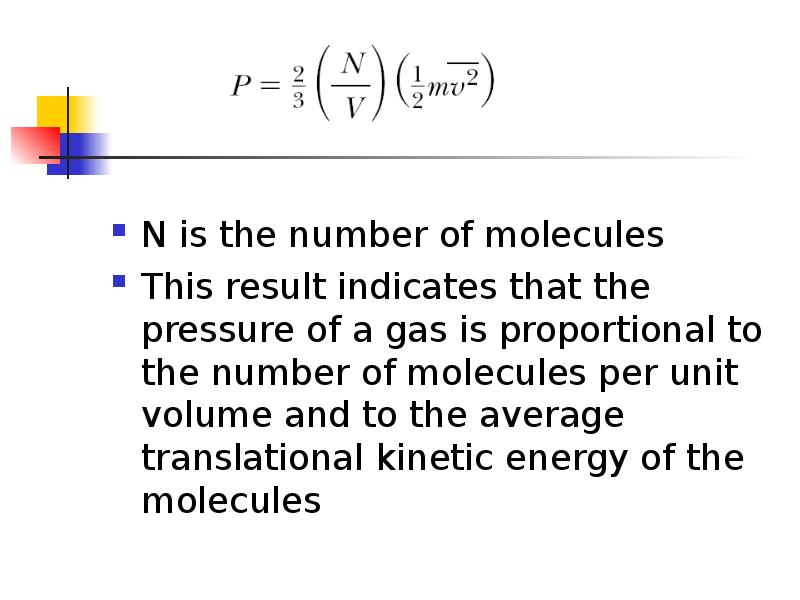Описание слайда:
N is the number of molecules This result indicates that the pressure of a gas is proportional to the number of molecules per unit volume and to the average translational kinetic energy of the molecules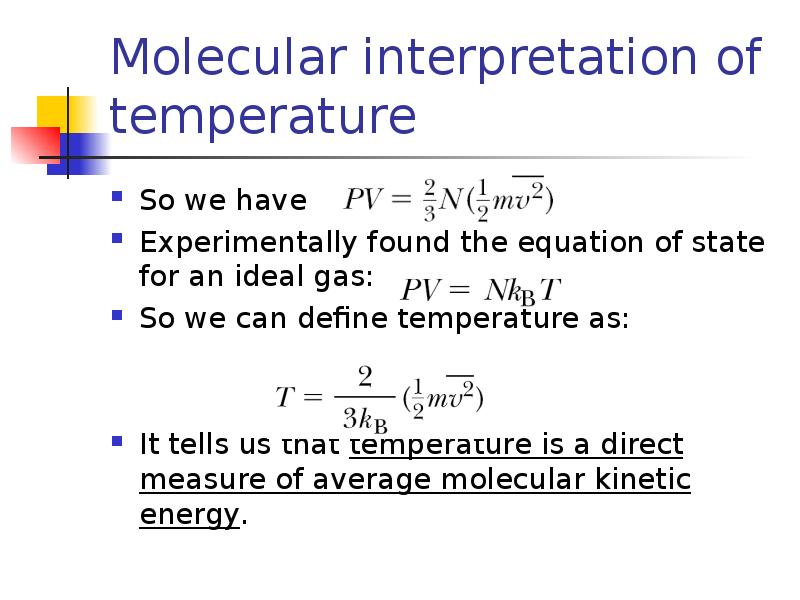Описание слайда:
Molecular interpretation of temperature So we have Experimentally found the equation of state for an ideal gas: So we can define temperature as: It tells us that temperature is a direct measure of average molecular kinetic energy.Описание слайда:
Theorem of equipartition of energy We can transform the last expression into Generalization of this result is known as the theorem of equipartition of energy: Each degree of freedom contributes to the energy of a system, where possible degrees of freedom in addition to translation arise from rotation and vibration of molecules.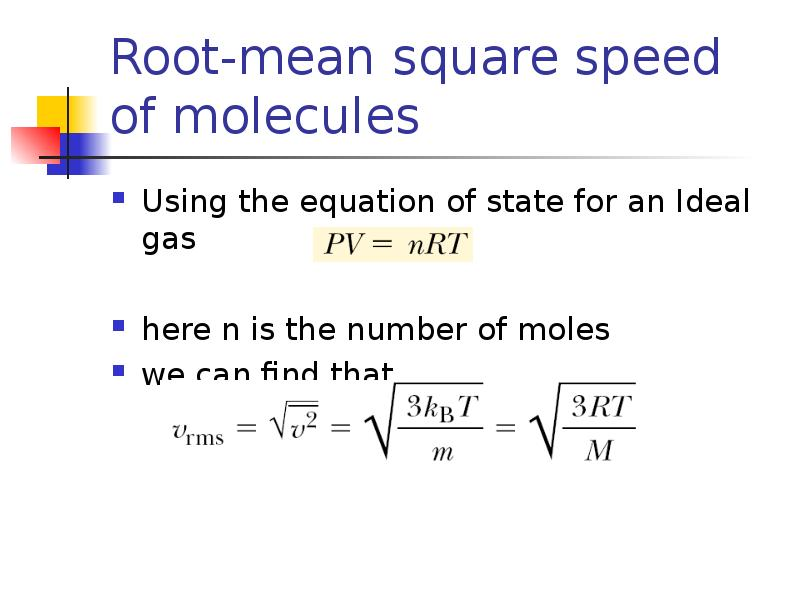Описание слайда:
Root-mean square speed of molecules Using the equation of state for an Ideal gas here n is the number of moles we can find that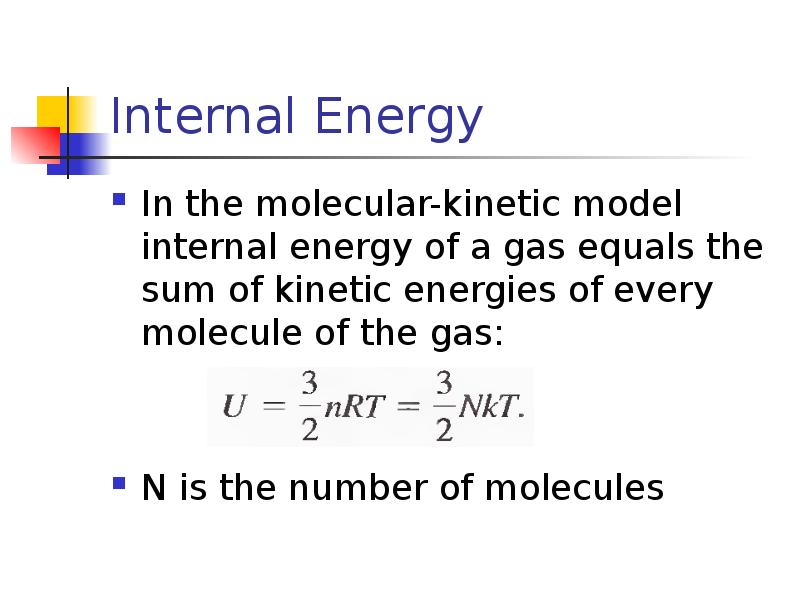Описание слайда:
Internal Energy In the molecular-kinetic model internal energy of a gas equals the sum of kinetic energies of every molecule of the gas: N is the number of molecules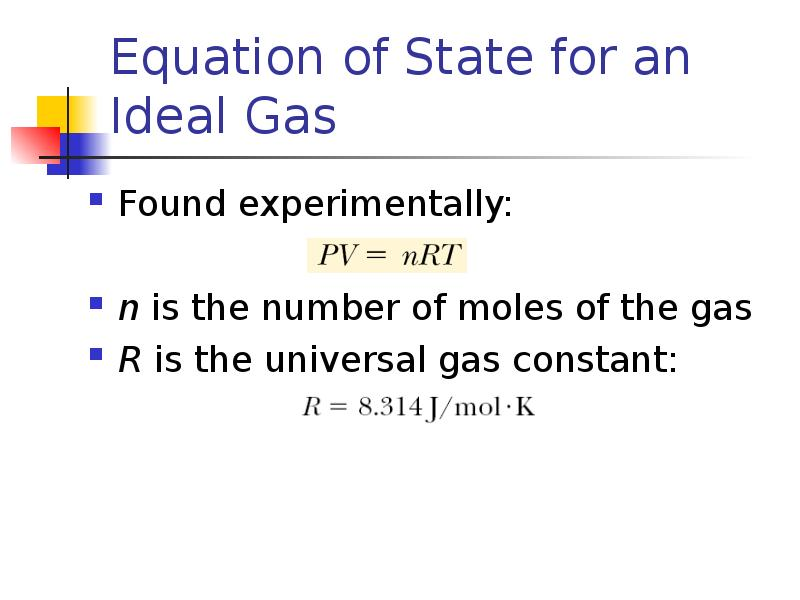Описание слайда:
Equation of State for an Ideal Gas Found experimentally: n is the number of moles of the gas R is the universal gas constant: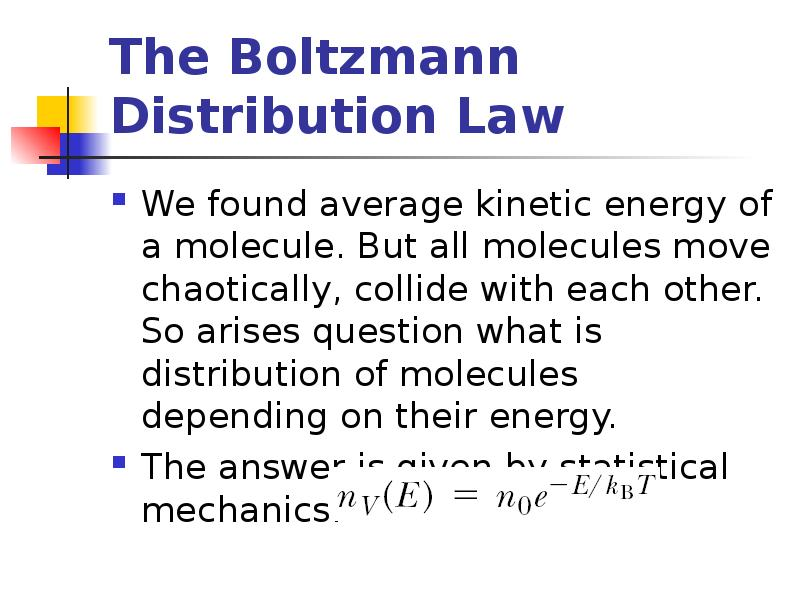Описание слайда:
The Boltzmann Distribution Law We found average kinetic energy of a molecule. But all molecules move chaotically, collide with each other. So arises question what is distribution of molecules depending on their energy. The answer is given by statistical mechanics: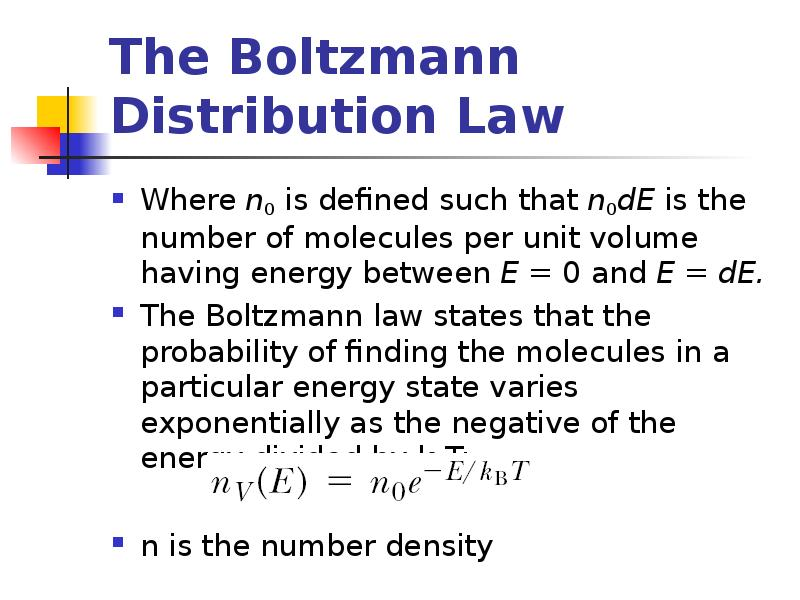Описание слайда:
The Boltzmann Distribution Law Where n0 is defined such that n0dE is the number of molecules per unit volume having energy between E = 0 and E = dE. The Boltzmann law states that the probability of finding the molecules in a particular energy state varies exponentially as the negative of the energy divided by kBT: n is the number density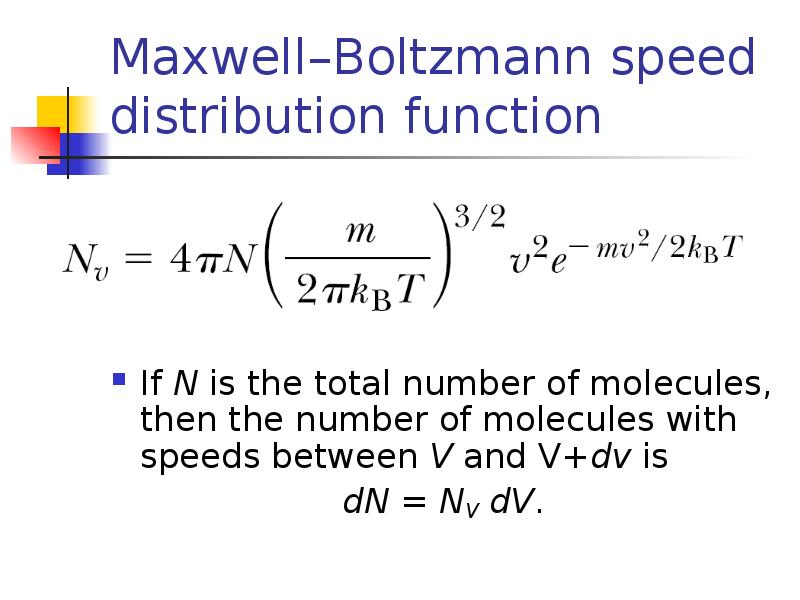Описание слайда:
Maxwell–Boltzmann speed distribution function If N is the total number of molecules, then the number of molecules with speeds between V and V+dv is dN = NV dV.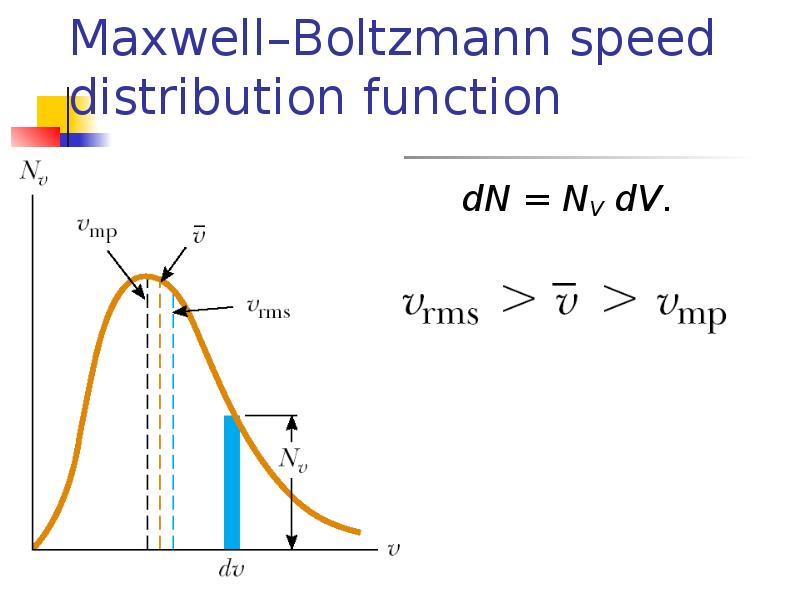Описание слайда:
Maxwell–Boltzmann speed distribution function dN = NV dV.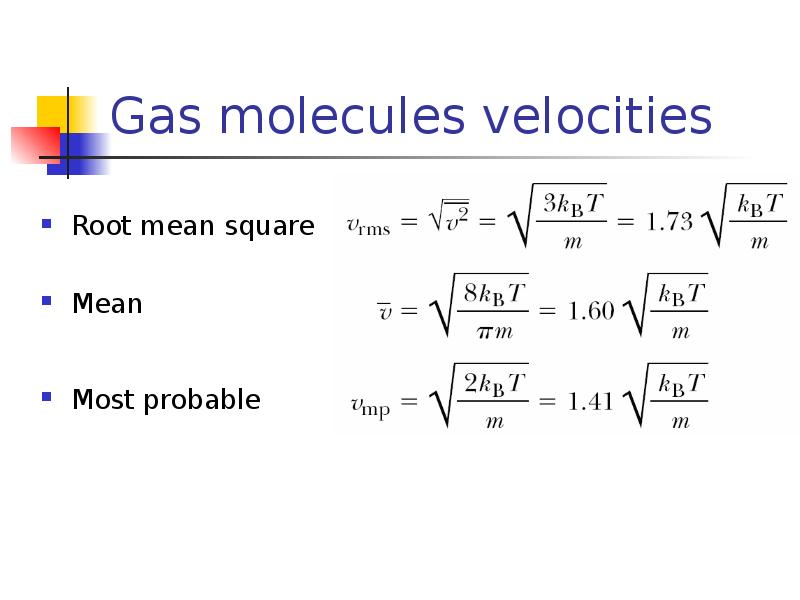Описание слайда:
Gas molecules velocities Root mean square Mean Most probable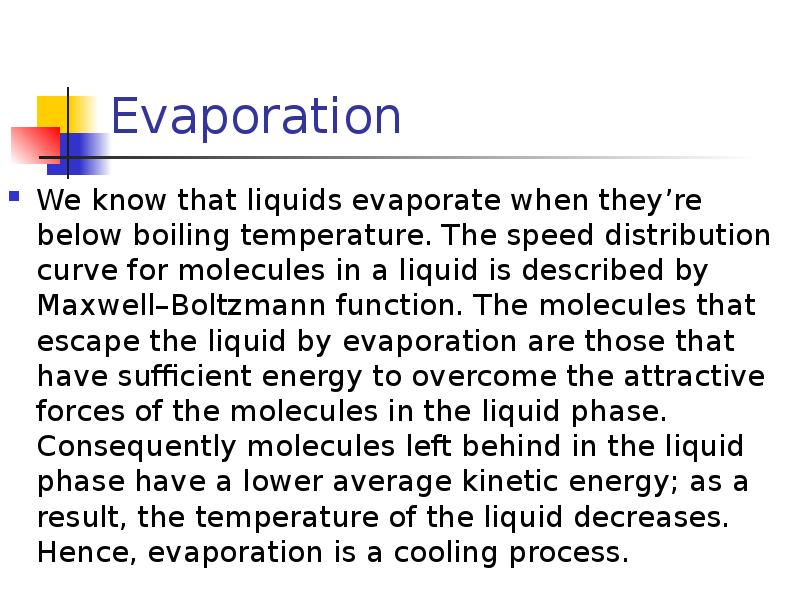Описание слайда:
Evaporation We know that liquids evaporate when they’re below boiling temperature. The speed distribution curve for molecules in a liquid is described by Maxwell–Boltzmann function. The molecules that escape the liquid by evaporation are those that have sufficient energy to overcome the attractive forces of the molecules in the liquid phase. Consequently molecules left behind in the liquid phase have a lower average kinetic energy; as a result, the temperature of the liquid decreases. Hence, evaporation is a cooling process.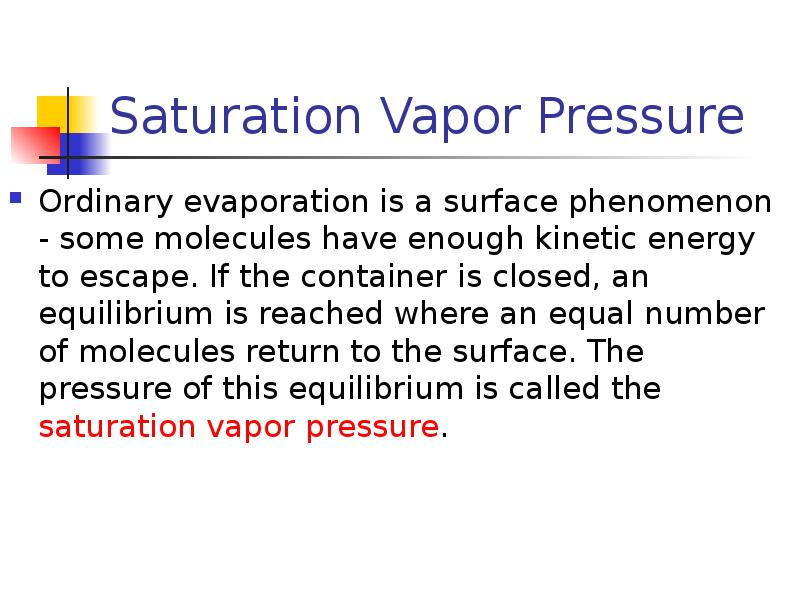Описание слайда:
Saturation Vapor Pressure Ordinary evaporation is a surface phenomenon - some molecules have enough kinetic energy to escape. If the container is closed, an equilibrium is reached where an equal number of molecules return to the surface. The pressure of this equilibrium is called the saturation vapor pressure.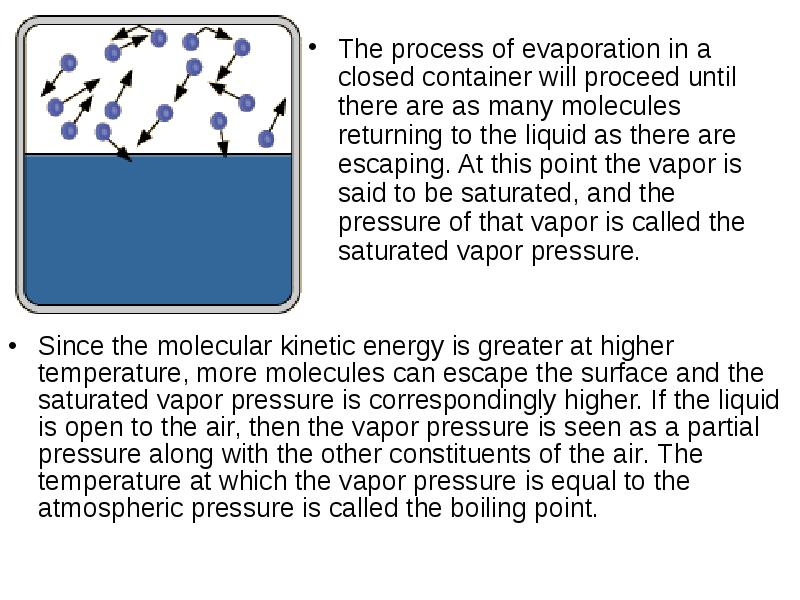Описание слайда:
The process of evaporation in a closed container will proceed until there are as many molecules returning to the liquid as there are escaping. At this point the vapor is said to be saturated, and the pressure of that vapor is called the saturated vapor pressure. The process of evaporation in a closed container will proceed until there are as many molecules returning to the liquid as there are escaping. At this point the vapor is said to be saturated, and the pressure of that vapor is called the saturated vapor pressure.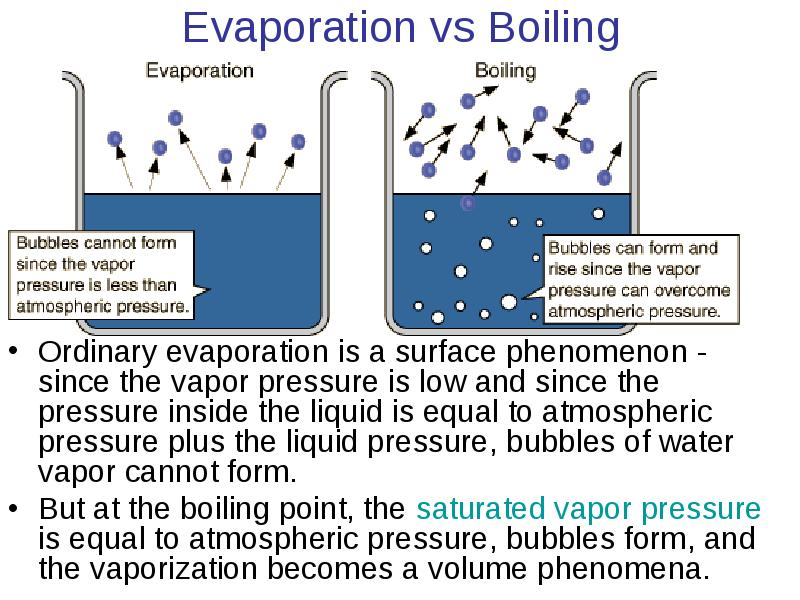Описание слайда:
Evaporation vs Boiling Ordinary evaporation is a surface phenomenon - since the vapor pressure is low and since the pressure inside the liquid is equal to atmospheric pressure plus the liquid pressure, bubbles of water vapor cannot form. But at the boiling point, the saturated vapor pressure is equal to atmospheric pressure, bubbles form, and the vaporization becomes a volume phenomena.Описание слайда:
Boiling Point The boiling point is defined as the temperature at which the saturated pressure of a liquid is equal to the surrounding atmospheric pressure. For water, the vapor pressure reaches the standard sea level atmospheric pressure of 760 mmHg at 100°C.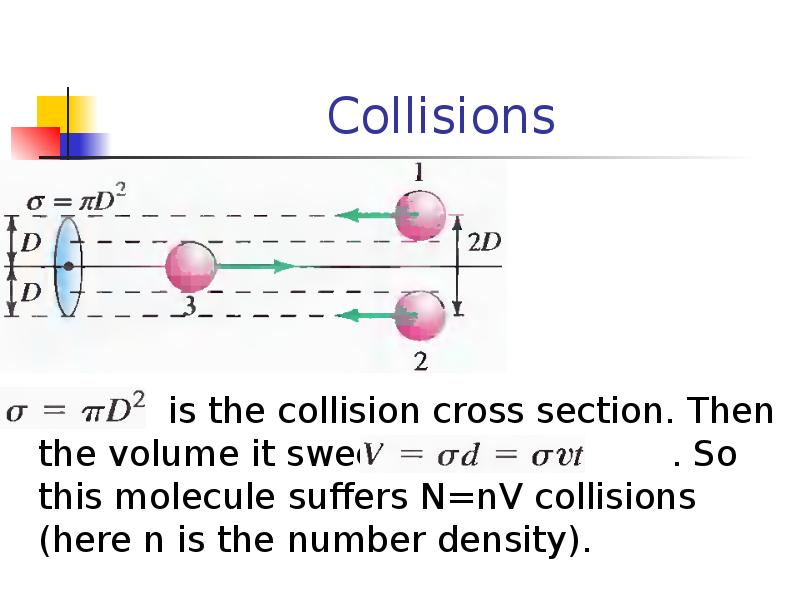Описание слайда:
Collisions is the collision cross section. Then the volume it sweeps is . So this molecule suffers N=nV collisions (here n is the number density).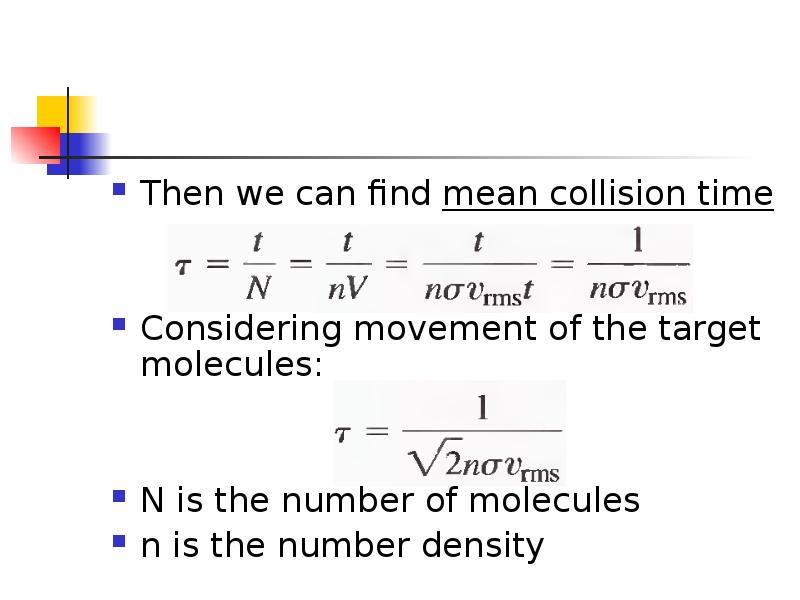Описание слайда:
Then we can find mean collision time Considering movement of the target molecules: N is the number of molecules n is the number density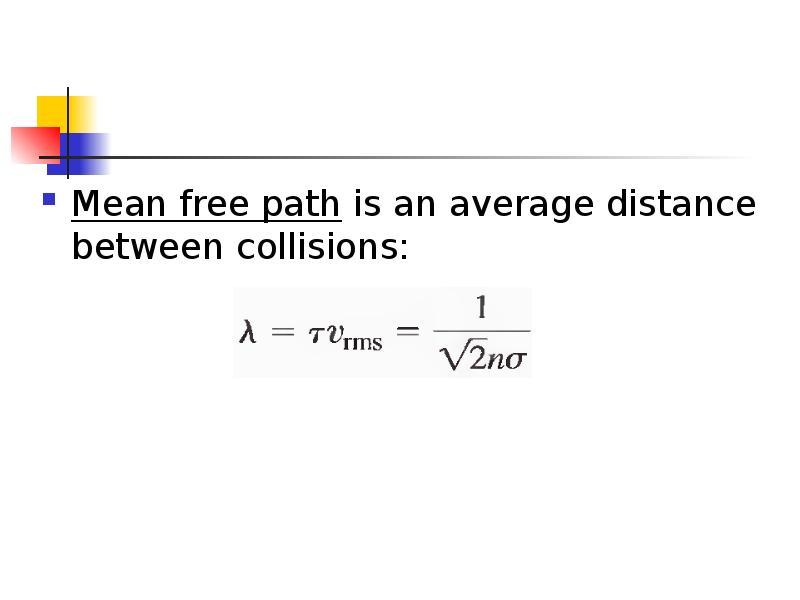Описание слайда:
Mean free path is an average distance between collisions: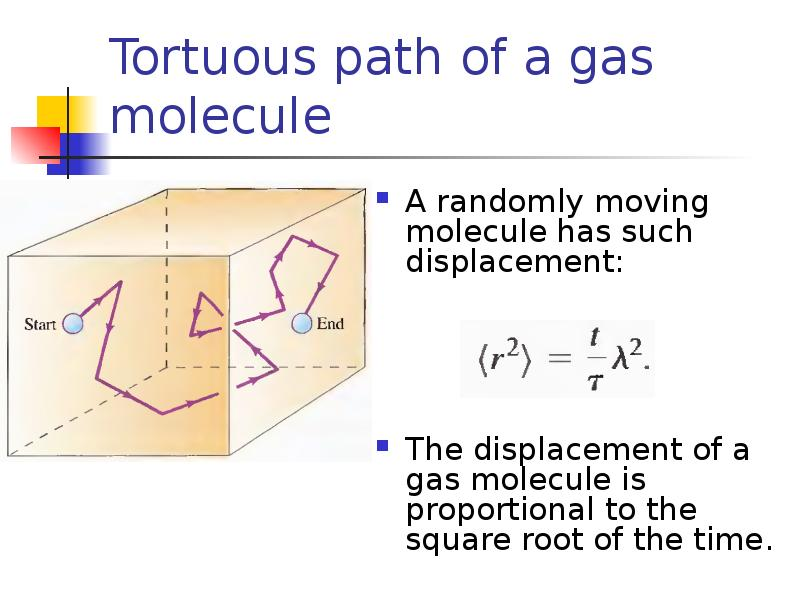Описание слайда:
Tortuous path of a gas molecule A randomly moving molecule has such displacement: The displacement of a gas molecule is proportional to the square root of the time.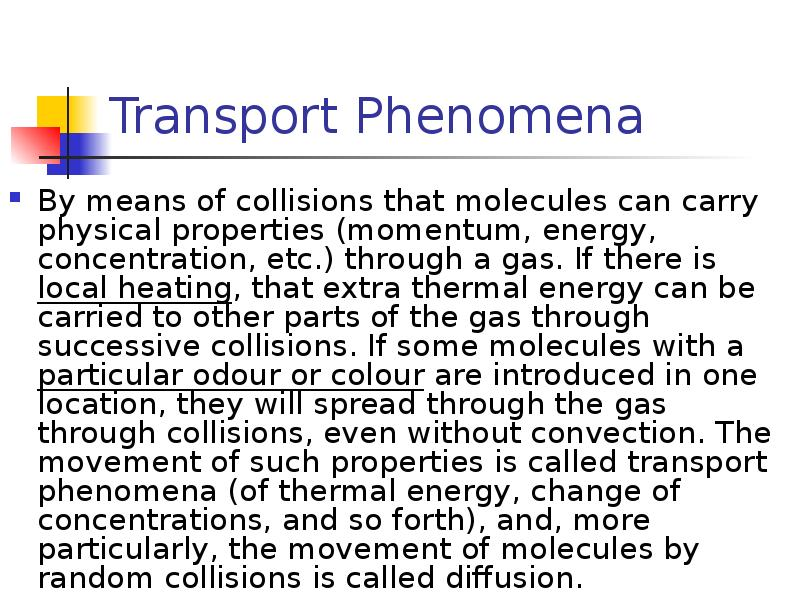Описание слайда:
Transport Phenomena By means of collisions that molecules can carry physical properties (momentum, energy, concentration, etc.) through a gas. If there is local heating, that extra thermal energy can be carried to other parts of the gas through successive collisions. If some molecules with a particular odour or colour are introduced in one location, they will spread through the gas through collisions, even without convection. The movement of such properties is called transport phenomena (of thermal energy, change of concentrations, and so forth), and, more particularly, the movement of molecules by random collisions is called diffusion.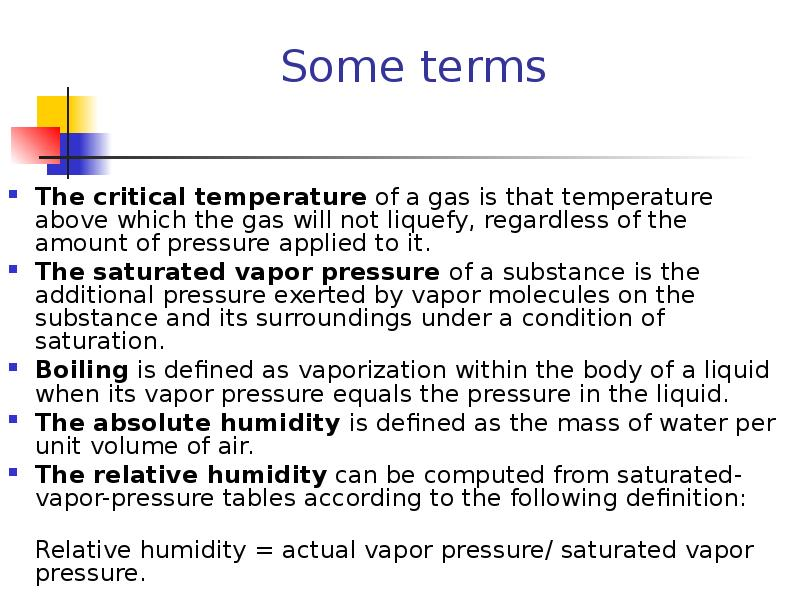Описание слайда:
Some terms The critical temperature of a gas is that temperature above which the gas will not liquefy, regardless of the amount of pressure applied to it. The saturated vapor pressure of a substance is the additional pressure exerted by vapor molecules on the substance and its surroundings under a condition of saturation. Boiling is defined as vaporization within the body of a liquid when its vapor pressure equals the pressure in the liquid. The absolute humidity is defined as the mass of water per unit volume of air. The relative humidity can be computed from saturated-vapor-pressure tables according to the following definition: Relative humidity = actual vapor pressure/ saturated vapor pressure.Описание слайда:
Van der Waals Gas n is the number of moles of the gas Term represents long-range attraction between molecules. Term b - the volume taken up by one mole of molecules. It accounts for the strong repulsion between molecules at a characteristic radius.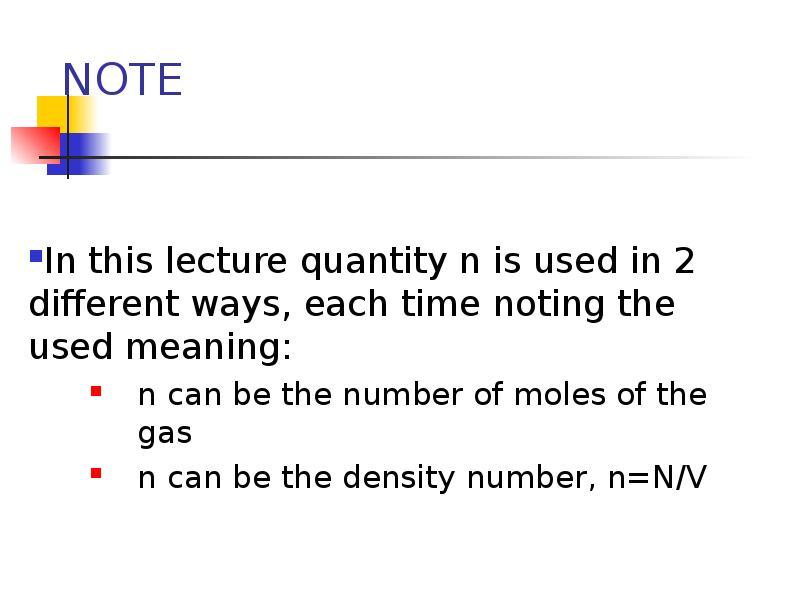Описание слайда:
NOTE In this lecture quantity n is used in 2 different ways, each time noting the used meaning: n can be the number of moles of the gas n can be the density number, n=N/V

Скачать презентацию на тему Molecular-kinetic theory of ideal gases можно ниже:

Похожие презентации